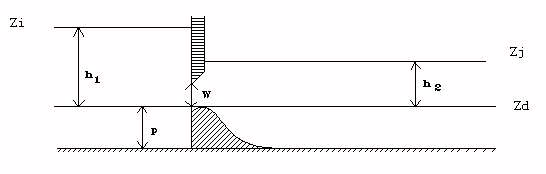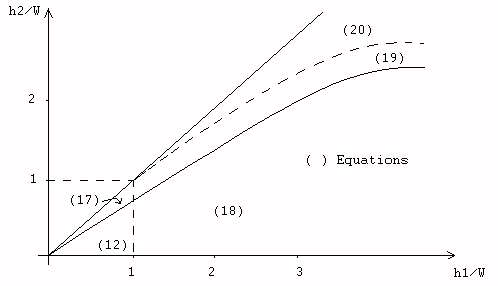# CEM88(D) : Weir / Orifice (low sill)## Weir - free flow

$$Q=\mu_f L \sqrt{2g} h_1^{3/2}$$

## Weir - submerged flow

$$Q=k_F \mu_F L \sqrt{2g} h_1^{3/2}$$

$$k_F$$ flow reduction coefficient in submerged flow. The flow reduction coefficient is a function of $$\frac{h_2}{h_1}$$ and the value $${\alpha}$$ of this ratio when switching from submerged flow to free flow. Flooding is achieved when $$\frac{h_2}{h_1} > \alpha$$. Variation law of $$k_F$$ was ajusted to experimental results ($$\alpha= 0.75$$).

Let's put $$x = \sqrt{1-\frac{h_2}{h_1}}$$:

• If $$x > 0.2 : k_F = 1 - \left(1 - \frac{x}{\sqrt{1-\alpha}}\right)^\beta$$
• If $$x \leq 0.2 : k_F = 5x \left(1 - \left(1 - \frac{0.2}{\sqrt{1-\alpha}} \right)^\beta \right)$$

With $$\beta = -2\alpha + 2.6$$, an equivalent free flow coefficient is calculated as above.

## Undershot gate - free flow

$$Q = L \sqrt{2g} \left(\mu h_1^{3/2} - \mu_1 (h_1 - W)^{3/2} \right)$$

Experimentally, the flow coefficient of a valve is found to increase with $$\frac{h_1}{W}$$. A law of variation of$$\mu$$ was adjusted, in the form of:

$$\mu = \mu_0 - \frac{0.08}{\frac{h_1}{W}}$$ with : $$\mu_0 \simeq 0.4$$

so $$\mu_1 = \mu_0 - \frac{0.08}{\frac{h_1}{W}-1}$$

To ensure continuity with the free surface for $$\frac{h1}{W} = 1$$, $$\mu_F = \mu_0 - 0.08$$ then has to be $$\mu_F = 0.32$$ for $$\mu_0 = 0.4$$

## Undershot gate - submerged flow

### Partially submerged flow

$$Q = L \sqrt{2g} \left[k_F \mu h_1^{3/2} - \mu_1 \left(h_1 - W \right)^{3/2} \right]$$

$$k_F$$ being the same as for free surface.

The switching from submerged flow to free flow was adjusted to experimental results, we then have a law like:

$$\alpha = 1 - 0.14 \frac{h_2}{W}$$

$$0.4 \leq \alpha \leq0.75$$

In order to ensure continuity with free surface operation, the free surface sumbmerged-free switch must therefore be for $$\alpha = 0.75$$ instead of $$2/3$$ in the orifice weir formulation.

### Totally submerged flow

$$Q = L \sqrt{2g} \left(k_F \mu h_1^{3/2} - k_{F1} \mu_1 \left(h_1 - W \right)^{3/2} \right)$$

Formulation of $$k_{F1}$$ is the same as the one of $$k_{F}$$ replacing $$h_2$$ with $$h_2-W$$ (and $$h_1$$ with $$h_1-W$$) for the calculation of coefficient $$x$$ and of $${\alpha}$$ (and therefore of $$k_{F1}$$).

Switching to totally submerged occurs for:

$$h_2 > \alpha_1 h_1 + (1 - \alpha_1) W$$

with : $$\alpha_1 = 1 - 0.14 \frac{h_2 - W}{W}$$

$$(\alpha_1 = \alpha (h_2-W))$$

Weir gate operation is represented by the above equations and Figure 20. Regardless of the type of flow under load, an equivalent free flow coefficient is calculated corresponding to a conventional free flow gate design:

$$C_F = \frac{Q}{L\sqrt{2g} W \sqrt{h_1}}$$

The default master coefficient for the device is a coefficient of $$C_G$$ usually close to $$0.6$$. We then transform it into $$\mu_0 = \frac{2}{3} C_G$$, which allows to calculate $$\mu$$ and $$\mu_1$$ of the equation of the free flow gate.

Note: it is possible to obtain $$C_F \neq C_G$$, even under free flow conditions, as long as the discharge coefficient increases with the ratio $$\frac{h_1}{W}$$.• (12) : Weir - free flow
• (19) : Orifice - partially submerged
• (17) : Weir - submerged
• (20) : Orifice - totally submerged
• (18) : Orifice - free flow

Figure 20. Weir - orifice